# Angles Worksheet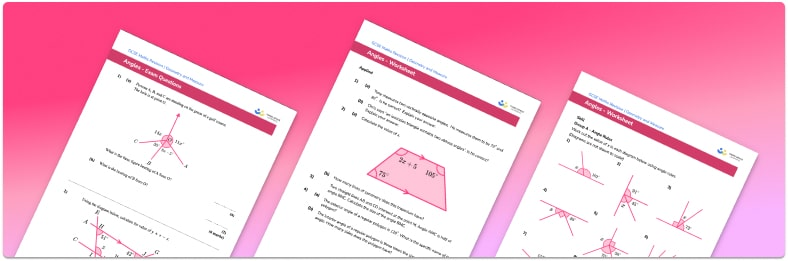• Section 1 of the angles worksheet contains 20+ skills-based angles questions, in 3 groups to support differentiation
• Section 2 contains 3 applied angles questions with a mix of worded problems and deeper problem solving questions
• Section 3 contains 3 foundation and higher level GCSE exam style angles questions
• Answers and a mark scheme for all questions are provided
• Questions follow variation theory with plenty of opportunities for students to work independently at their own level
• All questions created by fully qualified expert secondary maths teachers
• Suitable for GCSE maths revision for AQA, OCR and Edexcel exam boards

• This field is for validation purposes and should be left unchanged.

You can unsubscribe at any time (each email we send will contain an easy way to unsubscribe). To find out more about how we use your data, see our privacy policy.

### Angles at a glance

An angle is formed when two lines or rays intersect at a point. When measuring angles and drawing angles, we use a protractor and measure in degrees. When reading protractors, we give answers to the nearest whole degree.

It is important that students can identify angles such as acute, reflex, obtuse and right angles. We classify angles based on their size: an angle measuring less than 90° is an acute angle, greater than 90° but less than 180° is an obtuse angle, and greater than 180° is a reflex angle. An angle of exactly 90° is called a right angle.

Other angle facts that students need to know include: angles on a straight line and angles in a triangle add to 180°, angles in a quadrilateral add to 360°, and opposite angles in a parallelogram are equal. Students are expected to find the size of missing angles by selecting the appropriate angle fact and then using subtraction. Complementary angles are a pair of angles whose sum is 90°, and supplementary angles are a pair of angles whose sum is 180°.

When a pair of parallel lines is cut with an intersecting line (called a transversal), corresponding angles and alternate angles are formed.

Looking forward, students can then progress to additional angles worksheets and other geometry worksheets, for example an angles in polygons worksheet or volume and surface area of spheres worksheet.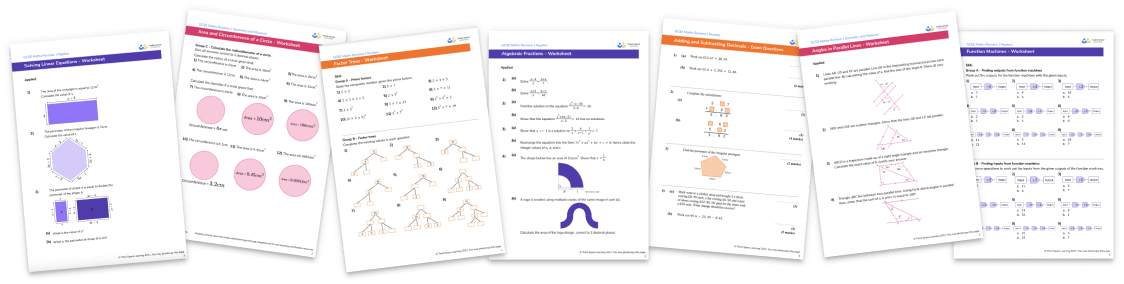For more teaching and learning support on Geometry our GCSE maths lessons provide step by step support for all GCSE maths concepts.

## Related worksheets

Types of Angles Worksheet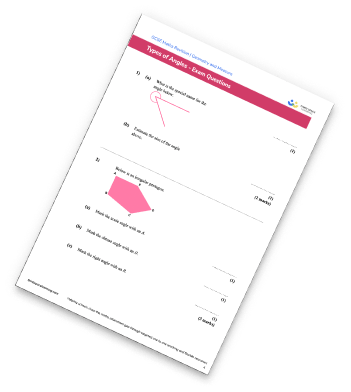Congruent Triangles Worksheet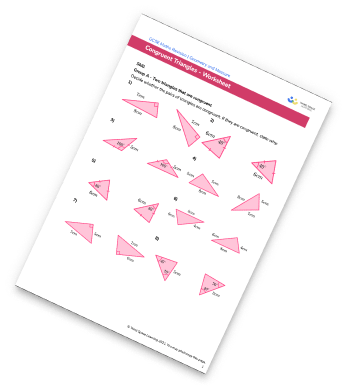Interior and Exterior Angles Worksheet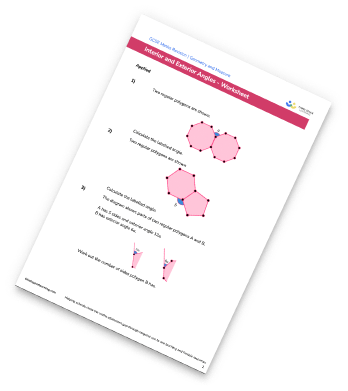Alternate Angles Worksheet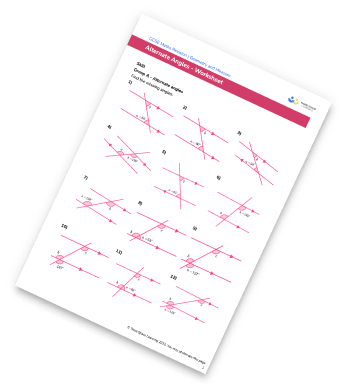## Do you have KS4 students who need more focused attention to succeed at GCSE?There will be students in your class who require individual attention to help them succeed in their maths GCSEs. In a class of 30, it’s not always easy to provide.

Help your students feel confident with exam-style questions and the strategies they’ll need to answer them correctly with our dedicated GCSE maths revision programme.

Lessons are selected to provide support where each student needs it most, and specially-trained GCSE maths tutors adapt the pitch and pace of each lesson. This ensures a personalised revision programme that raises grades and boosts confidence.

Find out more